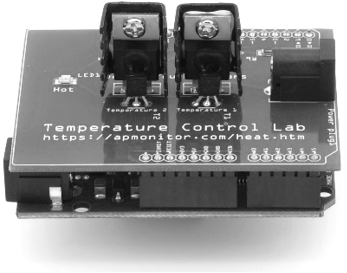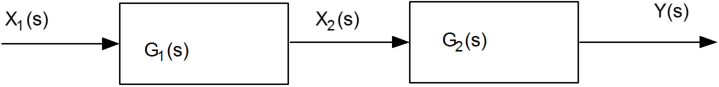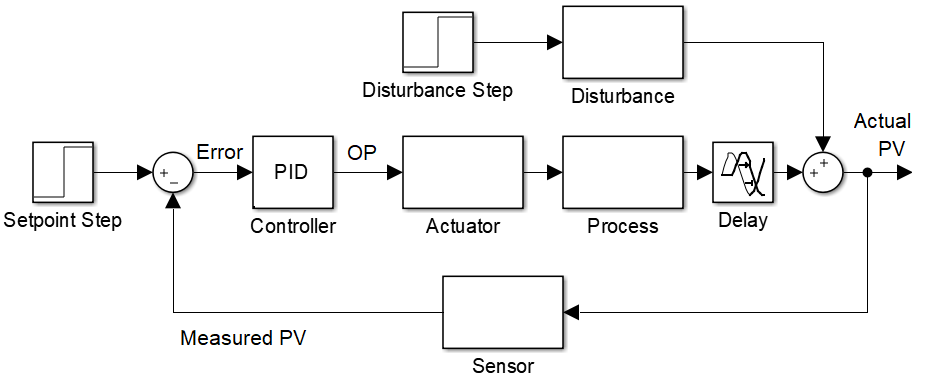## Transfer Functions in Block DiagramsOne source of transfer functions is from Balance Equations that relate inputs and outputs. Transfer functions are compact representations of dynamic systems and the differential equations become algebraic expressions that can be manipulated or combined with other expressions. The first step in creating a transfer function is to convert each term of a differential equation with a Laplace transform as shown in the table of Laplace transforms. A transfer function, G(s), relates an input, U(s), to an output, Y(s).

$$G(s) = \frac{Y(s)}{U(s)}$$

Do the following, assuming that q and V are constant:

1. Transform each equation in to the Laplace domain.
2. Find the transfer function between the specified variables.
3. Define the time constant and the gain for each transfer function in terms of the parameters given.

The following equations are linearized and variables are in deviation form with y'(t) = y(t) - y_{0}.

Exercise 1

Find {C(s)}/{C_i(s)}:

$$\frac{dc'(t)}{dt} = \frac{q}{V} c_i'(t) - \frac{q}{V} c'(t)$$

Exercise 2

Find {C(s)}/{C_i(s)}:

$$\frac{dc'(t)}{dt} = \frac{q}{V} c_i'(t) - \left(\frac{q}{V} + 2 k_2 \bar c\right) c'(t)$$

Exercise 3

The inlet temperature T_i(t) is a process disturbance and the heater Q(s) is adjusted to maintain the outlet temperature T(t) at a specified set point. Start with the following energy balance and find {T(s)}/{T_i(s)} and {T(s)}/{Q(s)}.

$$\frac{dT'(t)}{dt} = \frac{q}{V} \left(T_i'(t)-T'(t)\right) + \frac{Q'(t)}{\rho\,V\,C_p}$$

Determine if the two transfer functions are combined in parallel (additive) or series (multiplicative) and input the transfer functions in the appropriate boxes.

Transfer Functions in ParallelTransfer Functions in SeriesShow how the two transfer functions {T(s)}/{T_i(s)} and {T(s)}/{Q(s)} would fit into an overall control block diagram and label each block and the signal connections between each block. Cross off any blocks not needed for this problem.Solution